Documentation

## Modulation Classification with Deep Learning

This example shows how to use a convolutional neural network (CNN) for modulation classification. You generate synthetic, channel-impaired waveforms. Using the generated waveforms as training data, you train a CNN for modulation classification. You then test the CNN with software-defined radio (SDR) hardware and over-the-air signals.

### Predict Modulation Type Using CNN

The trained CNN in this example recognizes these eight digital and three analog modulation types:

• Binary phase shift keying (BPSK)

• Quadrature phase shift keying (QPSK)

• 8-ary phase shift keying (8-PSK)

• 16-ary quadrature amplitude modulation (16-QAM)

• 64-ary quadrature amplitude modulation (64-QAM)

• 4-ary pulse amplitude modulation (PAM4)

• Gaussian frequency shift keying (GFSK)

• Continuous phase frequency shift keying (CPFSK)

• Double sideband amplitude modulation (DSB-AM)

• Single sideband amplitude modulation (SSB-AM)

```modulationTypes = categorical(["BPSK", "QPSK", "8PSK", ... "16QAM", "64QAM", "PAM4", "GFSK", "CPFSK", ... "B-FM", "DSB-AM", "SSB-AM"]);```

First, load the trained network. For details on network training, see the Training a CNN section.

```load trainedModulationClassificationNetwork trainedNet```
```trainedNet = SeriesNetwork with properties: Layers: [28x1 nnet.cnn.layer.Layer] InputNames: {'Input Layer'} OutputNames: {'Output'} ```

The trained CNN takes 1024 channel-impaired samples and predicts the modulation type of each frame. Generate several BPSK frames that are impaired with Rician multipath fading, center frequency and sampling time drift, and AWGN. Use the `randi` function to generate random bits, the `pskmod` function to BPSK-modulate the bits, the `rcosdesign` function to design a square-root raised cosine pulse shaping filter, and the `filter` function to pulse shape the symbols. Then use the CNN to predict the modulation type of the frames.

```% Set the random number generator to a known state to be able to regenerate % the same frames every time the simulation is run rng(123456) % Random bits d = randi([0 1],1024,1); % BPSK modulation syms = pskmod(d,2); % Square-root raised cosine filter filterCoeffs = rcosdesign(0.35,4,8); tx = filter(filterCoeffs,1,upsample(syms,8)); % Channel channel = helperModClassTestChannel(... 'SampleRate',200e3, ... 'SNR',30, ... 'PathDelays',[0 1.8 3.4] / 200e3, ... 'AveragePathGains',[0 -2 -10], ... 'KFactor',4, ... 'MaximumDopplerShift',4, ... 'MaximumClockOffset',5, ... 'CenterFrequency',902e6); rx = channel(tx); % Plot transmitted and received signals scope = dsp.TimeScope(2,200e3,'YLimits',[-1 1],'ShowGrid',true,... 'LayoutDimensions',[2 1],'TimeSpan',45e-3); scope(tx,rx)```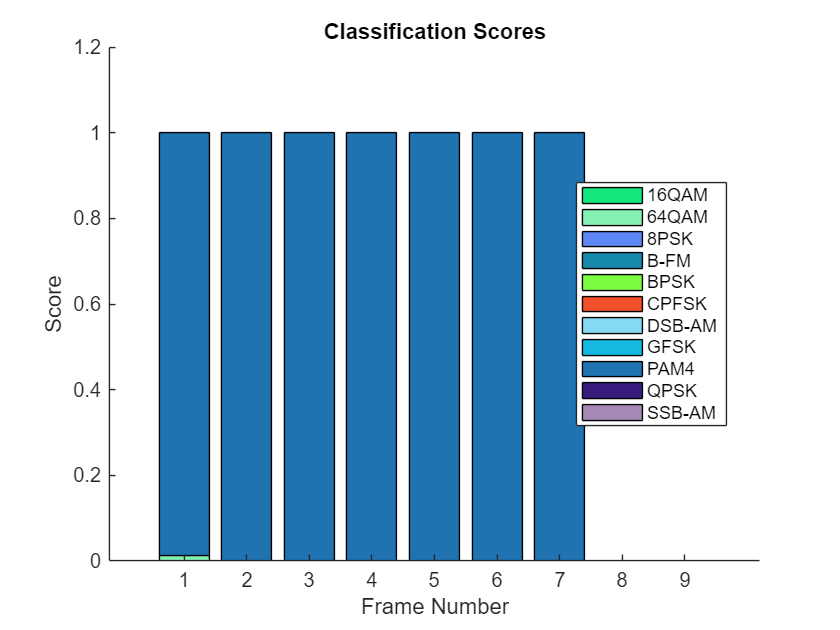```% Frame generation for classification unknownFrames = getNNFrames(rx,'Unknown'); % Classification [prediction1,score1] = classify(trainedNet,unknownFrames);```

Return the classifier predictions, which are analogous to hard decisions. The network correctly identifies the frames as BPSK frames. For details on the generation of the modulated signals, see Appendix: Modulators.

`prediction1`
```prediction1 = 7x1 categorical array BPSK BPSK BPSK BPSK BPSK BPSK BPSK ```

The classifier also returns a vector of scores for each frame. The score corresponds to the probability that each frame has the predicted modulation type. Plot the scores.

`plotScores(score1,modulationTypes)`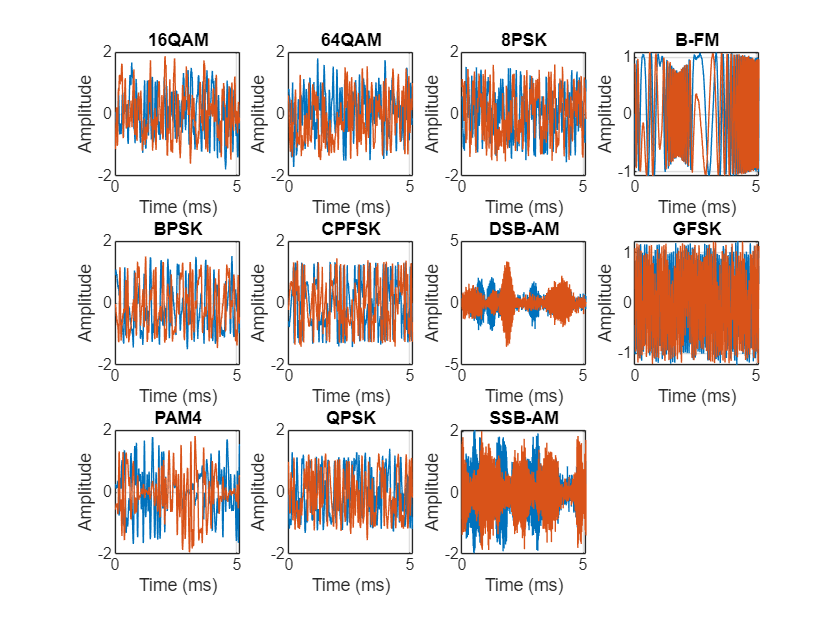Next, use the CNN to classify PAM4 frames.

```% Random bits d = randi([0 3], 1024, 1); % PAM4 modulation syms = pammod(d,4); % Square-root raised cosine filter filterCoeffs = rcosdesign(0.35, 4, 8); tx = filter(filterCoeffs, 1, upsample(syms,8)); % Channel rx = channel(tx); % Plot transmitted and received signals scope = dsp.TimeScope(2,200e3,'YLimits',[-2 2],'ShowGrid',true,... 'LayoutDimensions',[2 1],'TimeSpan',45e-3); scope(tx,rx)```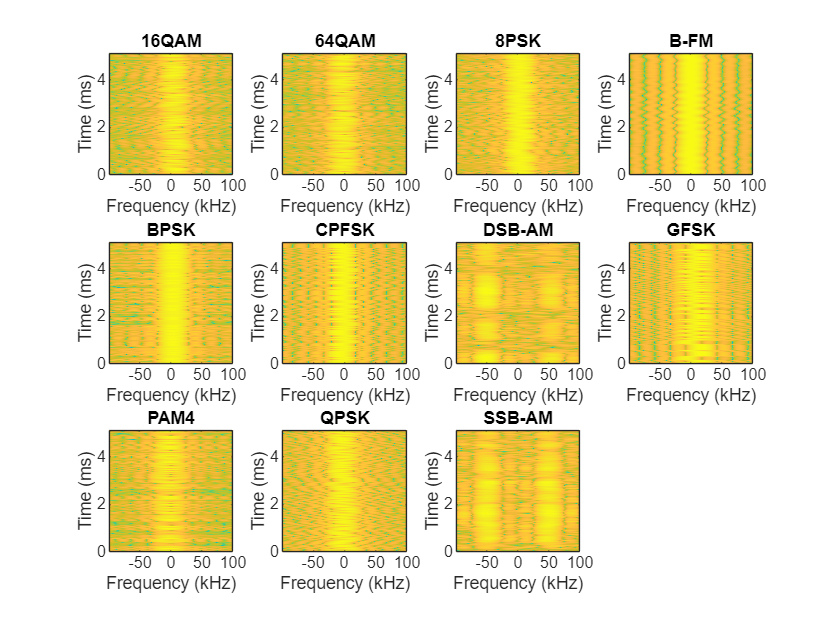```% Frame generation for classification unknownFrames = getNNFrames(rx,'Unknown'); % Classification [estimate2,score2] = classify(trainedNet,unknownFrames); estimate2```
```estimate2 = 7x1 categorical array PAM4 PAM4 PAM4 PAM4 PAM4 PAM4 PAM4 ```
`plotScores(score2,modulationTypes)`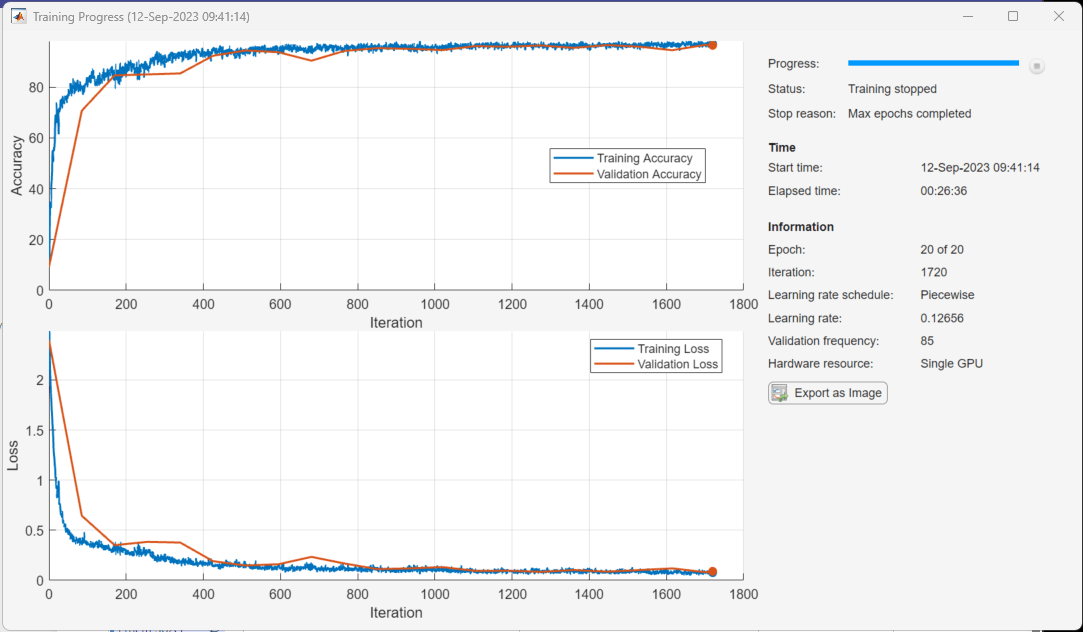Before we can use a CNN for modulation classification, or any other task, we first need to train the network with known (or labeled) data. The first part of this example shows how to use Communications Toolbox features, such as modulators, filters, and channel impairments, to generate synthetic training data. The second part focuses on defining, training, and testing the CNN for the task of modulation classification. The third part tests the network performance with over-the-air signals using software defined radio (SDR) platforms.

### Waveform Generation for Training

Generate 10,000 frames for each modulation type, where 80% is used for training, 10% is used for validation and 10% is used for testing. We use training and validation frames during the network training phase. Final classification accuracy is obtained using test frames. Each frame is 1024 samples long and has a sample rate of 200 kHz. For digital modulation types, eight samples represent a symbol. The network makes each decision based on single frames rather than on multiple consecutive frames (as in video). Assume a center frequency of 902 MHz and 100 MHz for the digital and analog modulation types, respectively.

To run this example quickly, use the trained network and generate a small number of training frames. To train the network on your computer, choose the "Train network now" option (i.e. set trainNow to true).

```trainNow =false; if trainNow == true numFramesPerModType = 10000; else numFramesPerModType = 500; end percentTrainingSamples = 80; percentValidationSamples = 10; percentTestSamples = 10; sps = 8; % Samples per symbol spf = 1024; % Samples per frame symbolsPerFrame = spf / sps; fs = 200e3; % Sample rate fc = [902e6 100e6]; % Center frequencies```

#### Create Channel Impairments

Pass each frame through a channel with

• AWGN

• Clock offset, resulting in center frequency offset and sampling time drift

Because the network in this example makes decisions based on single frames, each frame must pass through an independent channel.

#### AWGN

The channel adds AWGN with an SNR of 30 dB. Because the frames are normalized, the noise standard deviation, can be calculated as

```SNR = 30; std = sqrt(10.^(-SNR/10))```
```std = 0.0316 ```

Implement the channel using `comm.AWGNChannel`,

```awgnChannel = comm.AWGNChannel(... 'NoiseMethod', 'Signal to noise ratio (SNR)', ... 'SignalPower', 1, ... 'SNR', SNR)```
```awgnChannel = comm.AWGNChannel with properties: NoiseMethod: 'Signal to noise ratio (SNR)' SNR: 30 SignalPower: 1 RandomStream: 'Global stream' ```

#### Rician Multipath

The channel passes the signals through a Rician multipath fading channel using the `comm.RicianChannel` System object. Assume a delay profile of [0 1.8 3.4] samples with corresponding average path gains of [0 -2 -10] dB. The K-factor is 4 and the maximum Doppler shift is 4 Hz, which is equivalent to a walking speed at 902 MHz. Implement the channel with the following settings.

```multipathChannel = comm.RicianChannel(... 'SampleRate', fs, ... 'PathDelays', [0 1.8 3.4]/fs, ... 'AveragePathGains', [0 -2 -10], ... 'KFactor', 4, ... 'MaximumDopplerShift', 4)```
```multipathChannel = comm.RicianChannel with properties: SampleRate: 200000 PathDelays: [0 9.0000e-06 1.7000e-05] AveragePathGains: [0 -2 -10] NormalizePathGains: true KFactor: 4 DirectPathDopplerShift: 0 DirectPathInitialPhase: 0 MaximumDopplerShift: 4 DopplerSpectrum: [1x1 struct] Show all properties ```

#### Clock Offset

Clock offset occurs because of the inaccuracies of internal clock sources of transmitters and receivers. Clock offset causes the center frequency, which is used to downconvert the signal to baseband, and the digital-to-analog converter sampling rate to differ from the ideal values. The channel simulator uses the clock offset factor $\mathit{C}$, expressed as $\mathit{C}=1+\frac{{\Delta }_{\mathrm{clock}}}{{10}^{6}}$, where ${\Delta }_{\mathrm{clock}}$ is the clock offset. For each frame, the channel generates a random ${\Delta }_{\mathrm{clock}}$ value from a uniformly distributed set of values in the range [${-\mathrm{max}\Delta }_{\mathrm{clock}}$ ${\mathrm{max}\Delta }_{\mathrm{clock}}$], where ${\mathrm{max}\Delta }_{\mathrm{clock}}$ is the maximum clock offset. Clock offset is measured in parts per million (ppm). For this example, assume a maximum clock offset of 5 ppm.

```maxDeltaOff = 5; deltaOff = (rand()*2*maxDeltaOff) - maxDeltaOff; C = 1 + (deltaOff/1e6);```

Frequency Offset

Subject each frame to a frequency offset based on clock offset factor $\mathit{C}$ and the center frequency. Implemented the channel using `comm.PhaseFrequencyOffset`.

```offset = -(C-1)*fc(1); frequencyShifter = comm.PhaseFrequencyOffset(... 'SampleRate', fs, ... 'FrequencyOffset', offset)```
```frequencyShifter = comm.PhaseFrequencyOffset with properties: PhaseOffset: 0 FrequencyOffsetSource: 'Property' FrequencyOffset: -2.4386e+03 SampleRate: 200000 ```

Sampling Rate Offset

Subject each frame to a sampling rate offset based on clock offset factor $\mathit{C}$. Implement the channel using the `interp1` function to resample the frame at the new rate of $\mathit{C}×{\mathit{f}}_{\mathit{s}}$.

#### Combined Channel

Use the helperModClassTestChannel object to apply all three channel impairments to the frames.

```channel = helperModClassTestChannel(... 'SampleRate', fs, ... 'SNR', SNR, ... 'PathDelays', [0 1.8 3.4] / fs, ... 'AveragePathGains', [0 -2 -10], ... 'KFactor', 4, ... 'MaximumDopplerShift', 4, ... 'MaximumClockOffset', 5, ... 'CenterFrequency', 902e6)```
```channel = helperModClassTestChannel with properties: SNR: 30 CenterFrequency: 902000000 SampleRate: 200000 PathDelays: [0 9.0000e-06 1.7000e-05] AveragePathGains: [0 -2 -10] KFactor: 4 MaximumDopplerShift: 4 MaximumClockOffset: 5 ```

You can view basic information about the channel using the info object function.

`chInfo = info(channel)`
```chInfo = struct with fields: ChannelDelay: 6 MaximumFrequencyOffset: 4510 MaximumSampleRateOffset: 1 ```

#### Waveform Generation

Create a loop that generates channel-impaired frames for each modulation type and stores the frames with their corresponding labels in `frameStore`. Remove a random number of samples from the beginning of each frame to remove transients and to make sure that the frames have a random starting point with respect to the symbol boundaries.

```% Set the random number generator to a known state to be able to regenerate % the same frames every time the simulation is run rng(1235) tic numModulationTypes = length(modulationTypes); channelInfo = info(channel); frameStore = helperModClassFrameStore(... numFramesPerModType*numModulationTypes,spf,modulationTypes); transDelay = 50; for modType = 1:numModulationTypes fprintf('%s - Generating %s frames\n', ... datestr(toc/86400,'HH:MM:SS'), modulationTypes(modType)) numSymbols = (numFramesPerModType / sps); dataSrc = getSource(modulationTypes(modType), sps, 2*spf, fs); modulator = getModulator(modulationTypes(modType), sps, fs); if contains(char(modulationTypes(modType)), {'B-FM','DSB-AM','SSB-AM'}) % Analog modulation types use a center frequency of 100 MHz channel.CenterFrequency = 100e6; else % Digital modulation types use a center frequency of 902 MHz channel.CenterFrequency = 902e6; end for p=1:numFramesPerModType % Generate random data x = dataSrc(); % Modulate y = modulator(x); % Pass through independent channels rxSamples = channel(y); % Remove transients from the beginning, trim to size, and normalize frame = helperModClassFrameGenerator(rxSamples, spf, spf, transDelay, sps); % Add to frame store add(frameStore, frame, modulationTypes(modType)); end end```
```00:00:00 - Generating BPSK frames 00:00:03 - Generating QPSK frames 00:00:06 - Generating 8PSK frames 00:00:13 - Generating 16QAM frames 00:00:16 - Generating 64QAM frames 00:00:19 - Generating PAM4 frames 00:00:21 - Generating GFSK frames 00:00:24 - Generating CPFSK frames 00:00:26 - Generating B-FM frames 00:00:40 - Generating DSB-AM frames 00:00:43 - Generating SSB-AM frames ```

Next divide the frames into training, validation, and test data. By default, `frameStore` places I/Q baseband samples in rows in the output frames. The output frames have the size [2xspfx1xN], where the first row is in-phase samples and the second row is quadrature samples.

```[mcfsTraining,mcfsValidation,mcfsTest] = splitData(frameStore,... [percentTrainingSamples,percentValidationSamples,percentTestSamples]); [rxTraining,rxTrainingLabel] = get(mcfsTraining); [rxValidation,rxValidationLabel] = get(mcfsValidation); [rxTest,rxTestLabel] = get(mcfsTest);```
```% Plot the amplitude of the real and imaginary parts of the example frames % against the sample number plotTimeDomain(rxTest,rxTestLabel,modulationTypes,fs)```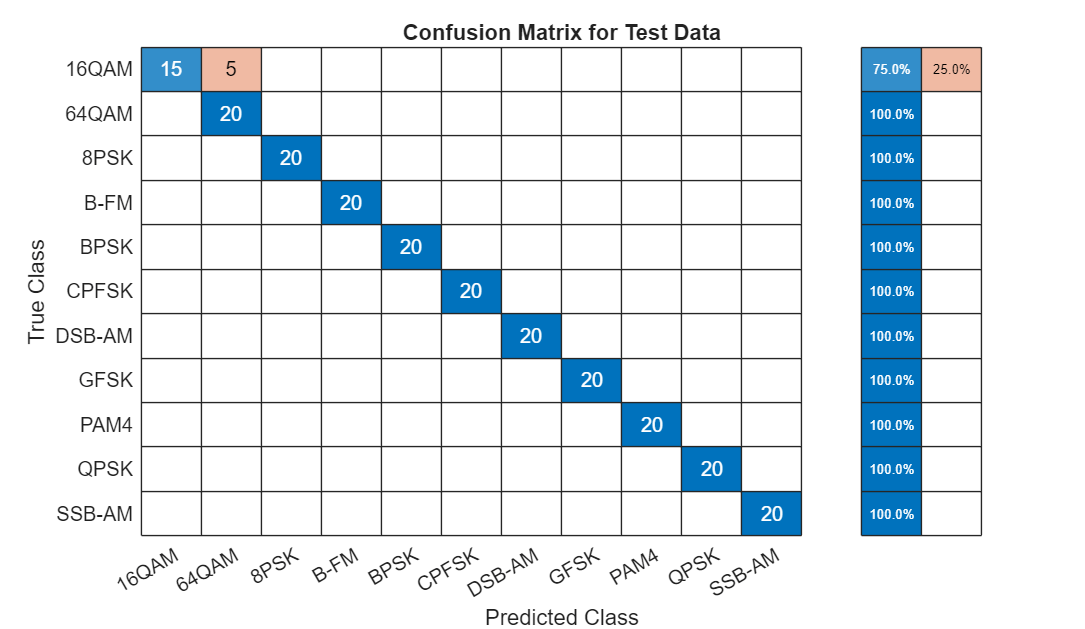```% Plot a spectrogram of the example frames plotSpectrogram(rxTest,rxTestLabel,modulationTypes,fs,sps)```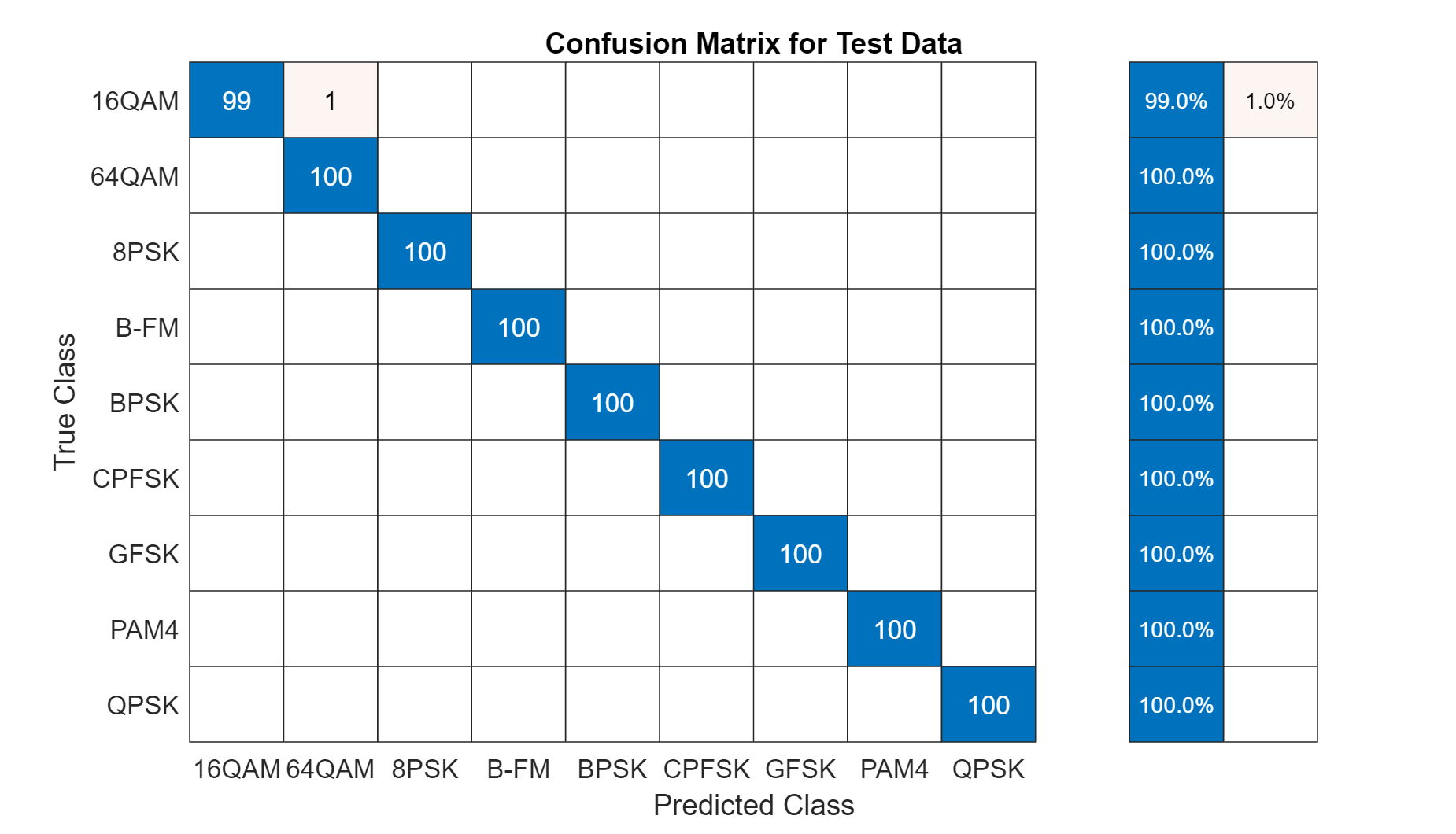Avoid class imbalance in your training data by ensuring a uniform distribution of labels (modulation types). Plot the label distributions to check if the generated labels are uniformly distributed.

```% Plot the label distributions figure subplot(3,1,1) histogram(rxTrainingLabel) title("Training Label Distribution") subplot(3,1,2) histogram(rxValidationLabel) title("Validation Label Distribution") subplot(3,1,3) histogram(rxTestLabel) title("Test Label Distribution")```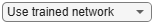### Train the CNN

This example uses a CNN that consists of six convolution layers and one fully connected layer. Each convolution layer except the last is followed by a batch normalization layer, rectified linear unit (ReLU) activation layer, and max pooling layer. In the last convolution layer, the max pooling layer is replaced with an average pooling layer. The output layer has softmax activation. For network design guidance, see Deep Learning Tips and Tricks (Deep Learning Toolbox).

```dropoutRate = 0.5; numModTypes = numel(modulationTypes); netWidth = 1; filterSize = [1 sps]; poolSize = [1 2]; modClassNet = [ imageInputLayer([2 spf 1], 'Normalization', 'none', 'Name', 'Input Layer') convolution2dLayer(filterSize, 16*netWidth, 'Padding', 'same', 'Name', 'CNN1') batchNormalizationLayer('Name', 'BN1') reluLayer('Name', 'ReLU1') maxPooling2dLayer(poolSize, 'Stride', [1 2], 'Name', 'MaxPool1') convolution2dLayer(filterSize, 24*netWidth, 'Padding', 'same', 'Name', 'CNN2') batchNormalizationLayer('Name', 'BN2') reluLayer('Name', 'ReLU2') maxPooling2dLayer(poolSize, 'Stride', [1 2], 'Name', 'MaxPool2') convolution2dLayer(filterSize, 32*netWidth, 'Padding', 'same', 'Name', 'CNN3') batchNormalizationLayer('Name', 'BN3') reluLayer('Name', 'ReLU3') maxPooling2dLayer(poolSize, 'Stride', [1 2], 'Name', 'MaxPool3') convolution2dLayer(filterSize, 48*netWidth, 'Padding', 'same', 'Name', 'CNN4') batchNormalizationLayer('Name', 'BN4') reluLayer('Name', 'ReLU4') maxPooling2dLayer(poolSize, 'Stride', [1 2], 'Name', 'MaxPool4') convolution2dLayer(filterSize, 64*netWidth, 'Padding', 'same', 'Name', 'CNN5') batchNormalizationLayer('Name', 'BN5') reluLayer('Name', 'ReLU5') maxPooling2dLayer(poolSize, 'Stride', [1 2], 'Name', 'MaxPool5') convolution2dLayer(filterSize, 96*netWidth, 'Padding', 'same', 'Name', 'CNN6') batchNormalizationLayer('Name', 'BN6') reluLayer('Name', 'ReLU6') averagePooling2dLayer([1 ceil(spf/32)], 'Name', 'AP1') fullyConnectedLayer(numModTypes, 'Name', 'FC1') softmaxLayer('Name', 'SoftMax') classificationLayer('Name', 'Output') ]```
```modClassNet = 28x1 Layer array with layers: 1 'Input Layer' Image Input 2x1024x1 images 2 'CNN1' Convolution 16 1x8 convolutions with stride [1 1] and padding 'same' 3 'BN1' Batch Normalization Batch normalization 4 'ReLU1' ReLU ReLU 5 'MaxPool1' Max Pooling 1x2 max pooling with stride [1 2] and padding [0 0 0 0] 6 'CNN2' Convolution 24 1x8 convolutions with stride [1 1] and padding 'same' 7 'BN2' Batch Normalization Batch normalization 8 'ReLU2' ReLU ReLU 9 'MaxPool2' Max Pooling 1x2 max pooling with stride [1 2] and padding [0 0 0 0] 10 'CNN3' Convolution 32 1x8 convolutions with stride [1 1] and padding 'same' 11 'BN3' Batch Normalization Batch normalization 12 'ReLU3' ReLU ReLU 13 'MaxPool3' Max Pooling 1x2 max pooling with stride [1 2] and padding [0 0 0 0] 14 'CNN4' Convolution 48 1x8 convolutions with stride [1 1] and padding 'same' 15 'BN4' Batch Normalization Batch normalization 16 'ReLU4' ReLU ReLU 17 'MaxPool4' Max Pooling 1x2 max pooling with stride [1 2] and padding [0 0 0 0] 18 'CNN5' Convolution 64 1x8 convolutions with stride [1 1] and padding 'same' 19 'BN5' Batch Normalization Batch normalization 20 'ReLU5' ReLU ReLU 21 'MaxPool5' Max Pooling 1x2 max pooling with stride [1 2] and padding [0 0 0 0] 22 'CNN6' Convolution 96 1x8 convolutions with stride [1 1] and padding 'same' 23 'BN6' Batch Normalization Batch normalization 24 'ReLU6' ReLU ReLU 25 'AP1' Average Pooling 1x32 average pooling with stride [1 1] and padding [0 0 0 0] 26 'FC1' Fully Connected 11 fully connected layer 27 'SoftMax' Softmax softmax 28 'Output' Classification Output crossentropyex ```

Use the `analyzeNetwork` function to display an interactive visualization of the network architecture, detect errors and issues with the network, and get detailed information about the network layers. This network has 98,323 learnables.

`analyzeNetwork(modClassNet)`Next configure `TrainingOptionsSGDM` to use an SGDM solver with a mini-batch size of 256. Set the maximum number of epochs to 12, since a larger number of epochs provides no further training advantage. Train the network on a GPU by setting the execution environment to `'gpu'`. Set the initial learning rate to $2\mathit{x}{10}^{-2}$. Reduce the learning rate by a factor of 10 every 9 epochs. Set `'Plots'` to '`training-progress'` to plot the training progress. On an NVIDIA Titan Xp GPU, the network takes approximately 25 minutes to train. .

```maxEpochs = 12; miniBatchSize = 256; validationFrequency = floor(numel(rxTrainingLabel)/miniBatchSize); options = trainingOptions('sgdm', ... 'InitialLearnRate',2e-2, ... 'MaxEpochs',maxEpochs, ... 'MiniBatchSize',miniBatchSize, ... 'Shuffle','every-epoch', ... 'Plots','training-progress', ... 'Verbose',false, ... 'ValidationData',{rxValidation,rxValidationLabel}, ... 'ValidationFrequency',validationFrequency, ... 'LearnRateSchedule', 'piecewise', ... 'LearnRateDropPeriod', 9, ... 'LearnRateDropFactor', 0.1, ... 'ExecutionEnvironment', 'gpu'); ```

Either train the network or use the already trained network. By default, this example uses the trained network.

```if trainNow == true fprintf('%s - Training the network\n', datestr(toc/86400,'HH:MM:SS')) trainedNet = trainNetwork(rxTraining,rxTrainingLabel,modClassNet,options); else load trainedModulationClassificationNetwork end```

As the plot of the training progress shows, the network converges in about 12 epochs to almost 90% accuracy.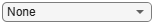Evaluate the trained network by obtaining the classification accuracy for the test frames. The results show that the network achieves about 90% accuracy for this group of waveforms.

`fprintf('%s - Classifying test frames\n', datestr(toc/86400,'HH:MM:SS'))`
```00:01:15 - Classifying test frames ```
```rxTestPred = classify(trainedNet,rxTest); testAccuracy = mean(rxTestPred == rxTestLabel); disp("Test accuracy: " + testAccuracy*100 + "%")```
```Test accuracy: 90.5455% ```

Plot the confusion matrix for the test frames. As the matrix shows, the network confuses 16-QAM and 64-QAM frames. This problem is expected since each frame carries only 128 symbols and 16-QAM is a subset of 64-QAM. The network also confuses QPSK and 8-PSK frames, since the constellations of these modulation types look similar once phase-rotated due to the fading channel and frequency offset.

```figure cm = confusionchart(rxTestLabel, rxTestPred); cm.Title = 'Confusion Matrix for Test Data'; cm.RowSummary = 'row-normalized'; cm.Parent.Position = [cm.Parent.Position(1:2) 740 424];```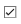#### I/Q as Pages

By default, `frameStore` places I/Q baseband samples in rows in a 2-D array. Since the convolution filters are of size [1xsps], the convolutional layers process in-phase and quadrature components independently. Only in the fully connected layer is information from the in-phase and quadrature components combined.

An alternative is to represent the I/Q samples as a 3-D array of size [1xSPFx2] that places the in-phase and quadrature components in the 3rd dimension (pages). This approach mixes the information in the I and Q even in the convolutional layers and makes better use of the phase information. Set the `'OutputFormat'` property of the frame store to `"IQAsPages"`, and size of the input layer to [1xSPFx2].

```% Put the data in [1xspfx2] format mcfsTraining.OutputFormat = "IQAsPages"; [rxTraining,rxTrainingLabel] = get(mcfsTraining); mcfsValidation.OutputFormat = "IQAsPages"; [rxValidation,rxValidationLabel] = get(mcfsValidation); mcfsTest.OutputFormat = "IQAsPages"; [rxTest,rxTestLabel] = get(mcfsTest); % Set the options options = trainingOptions('sgdm', ... 'InitialLearnRate',2e-2, ... 'MaxEpochs',maxEpochs, ... 'MiniBatchSize',miniBatchSize, ... 'Shuffle','every-epoch', ... 'Plots','training-progress', ... 'Verbose',false, ... 'ValidationData',{rxValidation,rxValidationLabel}, ... 'ValidationFrequency',validationFrequency, ... 'LearnRateSchedule', 'piecewise', ... 'LearnRateDropPeriod', 9, ... 'LearnRateDropFactor', 0.1, ... 'ExecutionEnvironment', 'gpu'); % Set the input layer input size to [1xspfx2] modClassNet(1) = ... imageInputLayer([1 spf 2], 'Normalization', 'none', 'Name', 'Input Layer'); ```

The following analysis of the network shows that each convolutional filter has a dimension of 1x8x2 that enables the convolutional layer to use both I and Q data in calculating one filter output.

```analyzeNetwork(modClassNet) ```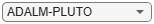```% Train or load the pretrained modified network if trainNow == true fprintf('%s - Training the network\n', datestr(toc/86400,'HH:MM:SS')) trainedNet = trainNetwork(rxTraining,rxTrainingLabel,modClassNet,options); else load trainedModulationClassificationNetwork2 end```

As the plot of the training progress shows, the network converges in about 12 epochs to more than 95% accuracy. Representing I/Q components as pages instead of rows can improve the accuracy of the network by about 5%.Evaluate the trained network by obtaining the classification accuracy for the test frames. The results show that the network achieves about 95% accuracy for this group of waveforms.

`fprintf('%s - Classifying test frames\n', datestr(toc/86400,'HH:MM:SS'))`
```00:01:24 - Classifying test frames ```
```rxTestPred = classify(trainedNet,rxTest); testAccuracy = mean(rxTestPred == rxTestLabel); disp("Test accuracy: " + testAccuracy*100 + "%")```
```Test accuracy: 95.2727% ```

Plot the confusion matrix for the test frames. As the matrix shows, representing I/Q components as pages instead of rows dramatically increases the ability of the network to accurately differentiate 16-QAM and 64-QAM frames and QPSK and 8-PSK frames.

```figure cm = confusionchart(rxTestLabel, rxTestPred); cm.Title = 'Confusion Matrix for Test Data'; cm.RowSummary = 'row-normalized'; cm.Parent.Position = [cm.Parent.Position(1:2) 740 424];```### Test with SDR

Test the performance of the trained network with over-the-air signals using the sdrTest function. To perform this test, you must have dedicated SDRs for transmission and reception. You can use two ADALM-PLUTO radios, or one ADALM-PLUTO radio for transmission and one USRP® radio for reception. You must install Communications Toolbox Support Package for ADALM-PLUTO Radio. If you are using a USRP® radio, you must also install Communications Toolbox Support Package for USRP® Radio. The `sdrTest` function uses the same modulation functions as used for generating the training signals, and then transmits them using an ADALM-PLUTO radio. Instead of simulating the channel, capture the channel-impaired signals using the SDR that is configured for signal reception (ADALM-PLUTO or USRP® radio). Use the trained network with the same `classify` function used previously to predict the modulation type. Running the next code segment produces a confusion matrix and prints out the test accuracy.

```radioPlatform ="ADALM-PLUTO"; switch radioPlatform case "ADALM-PLUTO" if isPlutoSDRInstalled() == true radios = findPlutoRadio(); if length(radios) >= 2 sdrTest(radios); else disp('Selected radios not found. Skipping over-the-air test.') end end case {"USRP B2xx","USRP X3xx","USRP N2xx"} if (isUSRPInstalled() == true) && (isPlutoSDRInstalled() == true) txRadio = findPlutoRadio(); rxRadio = findsdru(); switch radioPlatform case "USRP B2xx" idx = contains({rxRadio.Platform}, {'B200','B210'}); case "USRP X3xx" idx = contains({rxRadio.Platform}, {'X300','X310'}); case "USRP N2xx" idx = contains({rxRadio.Platform}, 'N200/N210/USRP2'); end rxRadio = rxRadio(idx); if (length(txRadio) >= 1) && (length(rxRadio) >= 1) sdrTest(rxRadio); else disp('Selected radios not found. Skipping over-the-air test.') end end end```

When using two stationary ADALM-PLUTO radios separated by about 2 feet, the network achieves 99% overall accuracy with the following confusion matrix. Results will vary based on experimental setup.### Further Exploration

It is possible to optimize the network parameters, such as number of filters, filter size, or optimize the network structure, such as adding more layers, using different activation layers, etc. to improve the accuracy.

Communication Toolbox provides many more modulation types and channel impairments. For more information see Modulation and Channel Models sections. You can also add standard specific signals with LTE Toolbox, WLAN Toolbox, and 5G Toolbox. You can also add radar signals with Phased Array System Toolbox.

Appendix: Modulators section provides the MATLAB functions used to generate modulated signals. You can also explore the following functions and System objects for more details:

### References

1. O'Shea, T. J., J. Corgan, and T. C. Clancy. "Convolutional Radio Modulation Recognition Networks." Preprint, submitted June 10, 2016. https://arxiv.org/abs/1602.04105

2. O'Shea, T. J., T. Roy, and T. C. Clancy. "Over-the-Air Deep Learning Based Radio Signal Classification." IEEE Journal of Selected Topics in Signal Processing. Vol. 12, Number 1, 2018, pp. 168–179.

3. Liu, X., D. Yang, and A. E. Gamal. "Deep Neural Network Architectures for Modulation Classification." Preprint, submitted January 5, 2018. https://arxiv.org/abs/1712.00443v3

### Appendix: Helper Functions

```function testAccuracy = sdrTest(radios) %sdrTest Test CNN performance with over-the-air signals % A = sdrTest sends test frames from an ADALM-PLUTO radio, receives using % an ADALM-PLUTO or USRP radio, performs classification with the trained % network and returns the overall classification accuracy. Transmitting % radio uses transmit-repeat capability to send the same waveform repeatedly % without loading the main loop. modulationTypes = categorical(["BPSK", "QPSK", "8PSK", ... "16QAM", "64QAM", "PAM4", "GFSK", "CPFSK", "B-FM"]); load trainedModulationClassificationNetwork2 trainedNet numFramesPerModType = 100; sps = 8; % Samples per symbol spf = 1024; % Samples per frame fs = 200e3; % Sample rate txRadio = sdrtx('Pluto'); txRadio.RadioID = 'usb:0'; txRadio.CenterFrequency = 902e6; txRadio.BasebandSampleRate = fs; if isfield(radios, 'Platform') radioPlatform = "USRP"; % Configure USRP radio as the receiver. rxRadio = comm.SDRuReceiver("Platform",radios(1).Platform); switch radios(1).Platform case {"B200","B210"} masterClockRate = 5e6; rxRadio.SerialNum = radios(1).SerialNum; case {"N200/N210/USRP2"} masterClockRate = 100e6; rxRadio.IPAddress = radios(1).IPAddress; case {"X300","X310"} masterClockRate = 120e6; rxRadio.IPAddress = radios(1).IPAddress; end rxRadio.MasterClockRate = masterClockRate; rxRadio.DecimationFactor = masterClockRate/fs; radioInfo = info(rxRadio); maximumGain = radioInfo.MaximumGain; minimumGain = radioInfo.MinimumGain; else radioPlatform = "PlutoSDR"; rxRadio = sdrrx('Pluto'); rxRadio.RadioID = 'usb:1'; rxRadio.BasebandSampleRate = fs; rxRadio.ShowAdvancedProperties = true; rxRadio.EnableQuadratureCorrection = false; rxRadio.GainSource = "Manual"; maximumGain = 73; minimumGain = -10; end rxRadio.SamplesPerFrame = spf; % Use burst mode with numFramesInBurst set to 1, so that each capture % (call to the receiver) will return an independent fresh frame even % though the radio overruns. rxRadio.EnableBurstMode = true; rxRadio.NumFramesInBurst = 1; rxRadio.OutputDataType = 'single'; % Display Tx and Rx radios txRadio rxRadio % Set random number generator to a known state to be able to regenerate % the same frames every time the simulation is run rng(1235) tic numModulationTypes = length(modulationTypes); txModType = repmat(modulationTypes(1),numModulationTypes*numFramesPerModType,1); estimatedModType = repmat(modulationTypes(1),numModulationTypes*numFramesPerModType,1); frameCnt = 1; for modType = 1:numModulationTypes fprintf('%s - Testing %s frames\n', ... datestr(toc/86400,'HH:MM:SS'), modulationTypes(modType)) dataSrc = getSource(modulationTypes(modType), sps, 2*spf, fs); modulator = getModulator(modulationTypes(modType), sps, fs); if contains(char(modulationTypes(modType)), {'B-FM'})... && (radioPlatform == "PlutoSDR") % Analog modulation types use a center frequency of 100 MHz txRadio.CenterFrequency = 100e6; rxRadio.CenterFrequency = 100e6; else % Digital modulation types use a center frequency of 902 MHz txRadio.CenterFrequency = 902e6; rxRadio.CenterFrequency = 902e6; end disp('Starting transmitter') x = dataSrc(); y = modulator(x); % Remove filter transients y = y(4*sps+1:end,1); maxVal = max(max(abs(real(y))), max(abs(imag(y)))); y = y *0.8/maxVal; % Download waveform signal to radio and repeatedly transmit it over the air transmitRepeat(txRadio, complex(y)); disp('Adjusting receiver gain') rxRadio.Gain = maximumGain; gainAdjusted = false; while ~gainAdjusted for p=1:20 rx = rxRadio(); end maxAmplitude = max([abs(real(rx)); abs(imag(rx))]); if (maxAmplitude < 0.8) || (rxRadio.Gain <= minimumGain) gainAdjusted = true; else rxRadio.Gain = rxRadio.Gain - 3; end end disp('Starting receiver and test') for p=1:numFramesPerModType rx = rxRadio(); frameEnergy = sum(abs(rx).^2); rx = rx / sqrt(frameEnergy); reshapedRx(1,:,1,1) = real(rx); reshapedRx(1,:,2,1) = imag(rx); % Classify txModType(frameCnt) = modulationTypes(modType); estimatedModType(frameCnt) = classify(trainedNet, reshapedRx); frameCnt = frameCnt + 1; % Pause for some time to get an independent channel. The pause duration % together with the processing time of a single loop must be greater % than the channel coherence time. Assume channel coherence time is less % than 0.1 seconds. pause(0.1) end disp('Releasing Tx radio') release(txRadio); testAccuracy = mean(txModType(1:frameCnt-1) == estimatedModType(1:frameCnt-1)); disp("Test accuracy: " + testAccuracy*100 + "%") end disp('Releasing Rx radio') release(rxRadio); testAccuracy = mean(txModType == estimatedModType); disp("Final test accuracy: " + testAccuracy*100 + "%") figure cm = confusionchart(txModType, estimatedModType); cm.Title = 'Confusion Matrix for Test Data'; cm.RowSummary = 'row-normalized'; cm.Parent.Position = [cm.Parent.Position(1:2) 740 424]; end function modulator = getModulator(modType, sps, fs) %getModulator Modulation function selector % MOD = getModulator(TYPE,SPS,FS) returns the modulator function handle % MOD based on TYPE. SPS is the number of samples per symbol and FS is % the sample rate. switch modType case "BPSK" modulator = @(x)bpskModulator(x,sps); case "QPSK" modulator = @(x)qpskModulator(x,sps); case "8PSK" modulator = @(x)psk8Modulator(x,sps); case "16QAM" modulator = @(x)qam16Modulator(x,sps); case "64QAM" modulator = @(x)qam64Modulator(x,sps); case "GFSK" modulator = @(x)gfskModulator(x,sps); case "CPFSK" modulator = @(x)cpfskModulator(x,sps); case "PAM4" modulator = @(x)pam4Modulator(x,sps); case "B-FM" modulator = @(x)bfmModulator(x, fs); case "DSB-AM" modulator = @(x)dsbamModulator(x, fs); case "SSB-AM" modulator = @(x)ssbamModulator(x, fs); end end function src = getSource(modType, sps, spf, fs) %getSource Source selector for modulation types % SRC = getSource(TYPE,SPS,SPF,FS) returns the data source % for the modulation type TYPE, with the number of samples % per symbol SPS, the number of samples per frame SPF, and % the sampling frequency FS. switch modType case {"BPSK","GFSK","CPFSK"} M = 2; src = @()randi([0 M-1],spf/sps,1); case {"QPSK","PAM4"} M = 4; src = @()randi([0 M-1],spf/sps,1); case "8PSK" M = 8; src = @()randi([0 M-1],spf/sps,1); case "16QAM" M = 16; src = @()randi([0 M-1],spf/sps,1); case "64QAM" M = 64; src = @()randi([0 M-1],spf/sps,1); case {"B-FM","DSB-AM","SSB-AM"} src = @()getAudio(spf,fs); end end function x = getAudio(spf,fs) %getAudio Audio source for analog modulation types % A = getAudio(SPF,FS) returns the audio source A, with the % number of samples per frame SPF, and the sample rate FS. persistent audioSrc audioRC if isempty(audioSrc) audioSrc = dsp.AudioFileReader('audio_mix_441.wav',... 'SamplesPerFrame',spf,'PlayCount',inf); audioRC = dsp.SampleRateConverter('Bandwidth',30e3,... 'InputSampleRate',audioSrc.SampleRate,... 'OutputSampleRate',fs); [~,decimFactor] = getRateChangeFactors(audioRC); audioSrc.SamplesPerFrame = ceil(spf / fs * audioSrc.SampleRate / decimFactor) * decimFactor; end x = audioRC(audioSrc()); x = x(1:spf,1); end function frames = getNNFrames(rx,modType) %getNNFrames Generate formatted frames for neural networks % F = getNNFrames(X,MODTYPE) formats the input X, into frames % that can be used with the neural network designed in this % example, and returns the frames in the output F. frames = helperModClassFrameGenerator(rx,1024,1024,32,8); frameStore = helperModClassFrameStore(10,1024,categorical({modType})); add(frameStore,frames,modType); frames = get(frameStore); end function plotScores(score,labels) %plotScores Plot classification scores of frames % plotScores(SCR,LABELS) plots the classification scores SCR as a stacked % bar for each frame. SCR is a matrix in which each row is the score for a % frame. co = [0.08 0.9 0.49; 0.52 0.95 0.70; 0.36 0.53 0.96; 0.09 0.54 0.67; 0.48 0.99 0.26; 0.95 0.31 0.17; 0.52 0.85 0.95; 0.08 0.72 0.88; 0.12 0.45 0.69; 0.22 0.11 0.49; 0.65 0.54 0.71]; figure; ax = axes('ColorOrder',co,'NextPlot','replacechildren'); bar(ax,[score; nan(2,11)],'stacked'); legend(categories(labels),'Location','best'); xlabel('Frame Number'); ylabel('Score'); title('Classification Scores') end function plotTimeDomain(rxTest,rxTestLabel,modulationTypes,fs) %plotTimeDomain Time domain plots of frames numRows = ceil(length(modulationTypes) / 4); spf = size(rxTest,2); t = 1000*(0:spf-1)/fs; if size(rxTest,1) == 2 IQAsRows = true; else IQAsRows = false; end for modType=1:length(modulationTypes) subplot(numRows, 4, modType); idxOut = find(rxTestLabel == modulationTypes(modType), 1); if IQAsRows rxI = rxTest(1,:,1,idxOut); rxQ = rxTest(2,:,1,idxOut); else rxI = rxTest(1,:,1,idxOut); rxQ = rxTest(1,:,2,idxOut); end plot(t,squeeze(rxI), '-'); grid on; axis equal; axis square hold on plot(t,squeeze(rxQ), '-'); grid on; axis equal; axis square hold off title(string(modulationTypes(modType))); xlabel('Time (ms)'); ylabel('Amplitude') end end function plotSpectrogram(rxTest,rxTestLabel,modulationTypes,fs,sps) %plotSpectrogram Spectrogram of frames if size(rxTest,1) == 2 IQAsRows = true; else IQAsRows = false; end numRows = ceil(length(modulationTypes) / 4); for modType=1:length(modulationTypes) subplot(numRows, 4, modType); idxOut = find(rxTestLabel == modulationTypes(modType), 1); if IQAsRows rxI = rxTest(1,:,1,idxOut); rxQ = rxTest(2,:,1,idxOut); else rxI = rxTest(1,:,1,idxOut); rxQ = rxTest(1,:,2,idxOut); end rx = squeeze(rxI) + 1i*squeeze(rxQ); spectrogram(rx,kaiser(sps),0,1024,fs,'centered'); title(string(modulationTypes(modType))); end h = gcf; delete(findall(h.Children, 'Type', 'ColorBar')) end function flag = isPlutoSDRInstalled %isPlutoSDRInstalled Check if ADALM-PLUTO Radio HSP is installed spkg = matlabshared.supportpkg.getInstalled; flag = ~isempty(spkg) && any(contains({spkg.Name},'ADALM-PLUTO','IgnoreCase',true)); end function flag = isUSRPInstalled %isUSRPInstalled Check if USRP Radio HSP is installed spkg = matlabshared.supportpkg.getInstalled; flag = ~isempty(spkg) && any(contains({spkg.Name},'USRP','IgnoreCase',true)); end```

### Appendix: Modulators

```function y = bpskModulator(x,sps) %bpskModulator BPSK modulator with pulse shaping % Y = bpskModulator(X,SPS) BPSK modulates the input X, and returns the % root-raised cosine pulse shaped signal Y. X must be a column vector % of values in the set [0 1]. The root-raised cosine filter has a % roll-off factor of 0.35 and spans four symbols. The output signal % Y has unit power. persistent filterCoeffs if isempty(filterCoeffs) filterCoeffs = rcosdesign(0.35, 4, sps); end % Modulate syms = pskmod(x,2); % Pulse shape y = filter(filterCoeffs, 1, upsample(syms,sps)); end function y = qpskModulator(x,sps) %qpskModulator QPSK modulator with pulse shaping % Y = qpskModulator(X,SPS) QPSK modulates the input X, and returns the % root-raised cosine pulse shaped signal Y. X must be a column vector % of values in the set [0 3]. The root-raised cosine filter has a % roll-off factor of 0.35 and spans four symbols. The output signal % Y has unit power. persistent filterCoeffs if isempty(filterCoeffs) filterCoeffs = rcosdesign(0.35, 4, sps); end % Modulate syms = pskmod(x,4,pi/4); % Pulse shape y = filter(filterCoeffs, 1, upsample(syms,sps)); end function y = psk8Modulator(x,sps) %psk8Modulator 8-PSK modulator with pulse shaping % Y = psk8Modulator(X,SPS) 8-PSK modulates the input X, and returns the % root-raised cosine pulse shaped signal Y. X must be a column vector % of values in the set [0 7]. The root-raised cosine filter has a % roll-off factor of 0.35 and spans four symbols. The output signal % Y has unit power. persistent filterCoeffs if isempty(filterCoeffs) filterCoeffs = rcosdesign(0.35, 4, sps); end % Modulate syms = pskmod(x,8); % Pulse shape y = filter(filterCoeffs, 1, upsample(syms,sps)); end function y = qam16Modulator(x,sps) %qam16Modulator 16-QAM modulator with pulse shaping % Y = qam16Modulator(X,SPS) 16-QAM modulates the input X, and returns the % root-raised cosine pulse shaped signal Y. X must be a column vector % of values in the set [0 15]. The root-raised cosine filter has a % roll-off factor of 0.35 and spans four symbols. The output signal % Y has unit power. persistent filterCoeffs if isempty(filterCoeffs) filterCoeffs = rcosdesign(0.35, 4, sps); end % Modulate and pulse shape syms = qammod(x,16,'UnitAveragePower',true); % Pulse shape y = filter(filterCoeffs, 1, upsample(syms,sps)); end function y = qam64Modulator(x,sps) %qam64Modulator 64-QAM modulator with pulse shaping % Y = qam64Modulator(X,SPS) 64-QAM modulates the input X, and returns the % root-raised cosine pulse shaped signal Y. X must be a column vector % of values in the set [0 63]. The root-raised cosine filter has a % roll-off factor of 0.35 and spans four symbols. The output signal % Y has unit power. persistent filterCoeffs if isempty(filterCoeffs) filterCoeffs = rcosdesign(0.35, 4, sps); end % Modulate syms = qammod(x,64,'UnitAveragePower',true); % Pulse shape y = filter(filterCoeffs, 1, upsample(syms,sps)); end function y = pam4Modulator(x,sps) %pam4Modulator PAM4 modulator with pulse shaping % Y = pam4Modulator(X,SPS) PAM4 modulates the input X, and returns the % root-raised cosine pulse shaped signal Y. X must be a column vector % of values in the set [0 3]. The root-raised cosine filter has a % roll-off factor of 0.35 and spans four symbols. The output signal % Y has unit power. persistent filterCoeffs amp if isempty(filterCoeffs) filterCoeffs = rcosdesign(0.35, 4, sps); amp = 1 / sqrt(mean(abs(pammod(0:3, 4)).^2)); end % Modulate syms = amp * pammod(x,4); % Pulse shape y = filter(filterCoeffs, 1, upsample(syms,sps)); end function y = gfskModulator(x,sps) %gfskModulator GFSK modulator % Y = gfskModulator(X,SPS) GFSK modulates the input X and returns the % signal Y. X must be a column vector of values in the set [0 1]. The % BT product is 0.35 and the modulation index is 1. The output signal % Y has unit power. persistent mod meanM if isempty(mod) M = 2; mod = comm.CPMModulator(... 'ModulationOrder', M, ... 'FrequencyPulse', 'Gaussian', ... 'BandwidthTimeProduct', 0.35, ... 'ModulationIndex', 1, ... 'SamplesPerSymbol', sps); meanM = mean(0:M-1); end % Modulate y = mod(2*(x-meanM)); end function y = cpfskModulator(x,sps) %cpfskModulator CPFSK modulator % Y = cpfskModulator(X,SPS) CPFSK modulates the input X and returns % the signal Y. X must be a column vector of values in the set [0 1]. % the modulation index is 0.5. The output signal Y has unit power. persistent mod meanM if isempty(mod) M = 2; mod = comm.CPFSKModulator(... 'ModulationOrder', M, ... 'ModulationIndex', 0.5, ... 'SamplesPerSymbol', sps); meanM = mean(0:M-1); end % Modulate y = mod(2*(x-meanM)); end function y = bfmModulator(x,fs) %bfmModulator Broadcast FM modulator % Y = bfmModulator(X,FS) broadcast FM modulates the input X and returns % the signal Y at the sample rate FS. X must be a column vector of % audio samples at the sample rate FS. The frequency deviation is 75 kHz % and the pre-emphasis filter time constant is 75 microseconds. persistent mod if isempty(mod) mod = comm.FMBroadcastModulator(... 'AudioSampleRate', fs, ... 'SampleRate', fs); end y = mod(x); end function y = dsbamModulator(x,fs) %dsbamModulator Double sideband AM modulator % Y = dsbamModulator(X,FS) double sideband AM modulates the input X and % returns the signal Y at the sample rate FS. X must be a column vector of % audio samples at the sample rate FS. The IF frequency is 50 kHz. y = ammod(x,50e3,fs); end function y = ssbamModulator(x,fs) %ssbamModulator Single sideband AM modulator % Y = ssbamModulator(X,FS) single sideband AM modulates the input X and % returns the signal Y at the sample rate FS. X must be a column vector of % audio samples at the sample rate FS. The IF frequency is 50 kHz. y = ssbmod(x,50e3,fs); end```